Next: Counting measurements in the Up: Uncertainty due to systematic Previous: Measuring two quantities with   Contents

## Indirect calibration

Let us use the result of the previous section to solve another typical problem of measurements. Suppose that after (or before, it doesn't matter) we have done the measurements of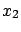and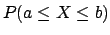and we have the final result, summarized in (), we know the exact'' value of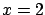(for example we perform the measurement on a reference). Let us call it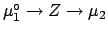. Will this information provide a better knowledge of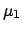? In principle yes: the difference betweenanddefines the systematic error (the true value of the zero''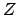). This error can then be subtracted fromto get a corrected value. Also the overall uncertainty ofshould change, intuitively it should'' decrease, since we are adding new information. But its value doesn't seem to be obvious, since the logical link betweenandis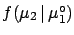.

The problem can be solved exactly using the concept of conditional probability density function[see ()-()). We get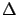(5.83)

The best value ofis shifted by an amount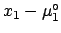, with respect to the measured value, which is not exactly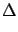, as was naï vely guessed, and the uncertainty depends on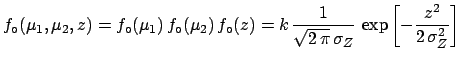,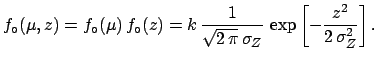and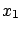. It is easy to be convinced that the exact result is more reasonable than the (suggested) first guess. Let us rewritein two different ways: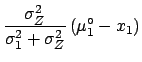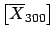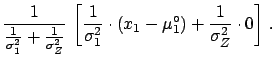(5.84)(5.85)

• Eq. () shows that one has to apply the correctiononly if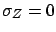. If insteadthere is no correction to be applied, since the instrument is perfectly calibrated. If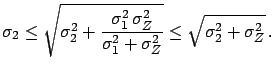the correction is half of the measured difference betweenand.
• Eq. () shows explicitly what is going on and why the result is consistent with the way we have modelled the uncertainties. In fact we have performed two independent calibrations: one of the offset and one of. The best estimate of the true value of the zero''is the weighted average of the two measured offsets.
• The new uncertainty of[see ()] is a combination ofand the uncertainty of the weighted average of the two offsets. Its value is smaller than it would be with only one calibration and, obviously, larger than that due to the sampling fluctuations alone: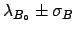(5.86)Next: Counting measurements in the Up: Uncertainty due to systematic Previous: Measuring two quantities with   Contents
Giulio D'Agostini 2003-05-15